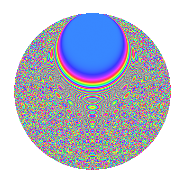# Properties

 Label 2009.2.yLevel 2009 Weight 2 Character orbit y Rep. character $$\chi_{2009}(197,\cdot)$$ Character field $$\Q(\zeta_{20})$$ Dimension 1112 Sturm bound 392

# Related objects

## Defining parameters

 Level: $$N$$ = $$2009 = 7^{2} \cdot 41$$ Weight: $$k$$ = $$2$$ Character orbit: $$[\chi]$$ = 2009.y (of order $$20$$ and degree $$8$$) Character conductor: $$\operatorname{cond}(\chi)$$ = $$41$$ Character field: $$\Q(\zeta_{20})$$ Sturm bound: $$392$$

## Dimensions

The following table gives the dimensions of various subspaces of $$M_{2}(2009, [\chi])$$.

Total New Old
Modular forms 1632 1192 440
Cusp forms 1504 1112 392
Eisenstein series 128 80 48

## Trace form

 $$1112q + 10q^{2} + 10q^{3} + 276q^{4} + 10q^{5} + 30q^{6} - 50q^{8} + O(q^{10})$$ $$1112q + 10q^{2} + 10q^{3} + 276q^{4} + 10q^{5} + 30q^{6} - 50q^{8} + 42q^{10} + 8q^{11} + 14q^{12} + 16q^{13} - 24q^{15} - 240q^{16} + 12q^{17} + 60q^{18} + 24q^{19} - 20q^{20} - 58q^{22} + 24q^{23} + 40q^{24} + 244q^{25} + 8q^{26} - 14q^{27} - 104q^{29} + 88q^{30} - 8q^{31} - 10q^{33} + 48q^{34} - 160q^{36} - 2q^{38} - 30q^{39} + 108q^{40} - 80q^{43} + 104q^{44} - 24q^{45} - 110q^{46} + 68q^{47} - 70q^{48} - 24q^{51} - 36q^{52} - 30q^{53} - 16q^{54} + 52q^{55} - 118q^{57} + 32q^{58} + 42q^{59} - 128q^{60} - 30q^{61} + 10q^{62} + 230q^{64} + 72q^{65} - 58q^{66} - 6q^{67} + 100q^{68} + 106q^{69} - 120q^{71} + 98q^{72} + 30q^{74} + 116q^{75} + 124q^{76} - 42q^{78} - 6q^{79} - 130q^{80} - 884q^{81} + 158q^{82} - 40q^{83} - 116q^{85} - 66q^{86} + 10q^{87} - 114q^{88} + 64q^{89} + 70q^{90} - 204q^{92} - 38q^{93} - 110q^{94} - 48q^{95} + 2q^{96} + 22q^{97} - 62q^{99} + O(q^{100})$$

## Decomposition of $$S_{2}^{\mathrm{new}}(2009, [\chi])$$ into irreducible Hecke orbits

The newforms in this space have not yet been added to the LMFDB.

## Decomposition of $$S_{2}^{\mathrm{old}}(2009, [\chi])$$ into lower level spaces

$$S_{2}^{\mathrm{old}}(2009, [\chi]) \cong$$ $$S_{2}^{\mathrm{new}}(41, [\chi])$$$$^{\oplus 3}$$$$\oplus$$$$S_{2}^{\mathrm{new}}(287, [\chi])$$$$^{\oplus 2}$$